Ch.11 - Liquids, Solids & Intermolecular ForcesWorksheetSee all chapters

# Unit Cell

See all sections
Sections
Molecular Polarity
Intermolecular Forces
Phase Diagram
Heating and Cooling Curves
Unit Cell
Clausius-Clapeyron Equation

Crystalline solids represent structures with well-organized patterns and shapes.

###### Crystalline Solids vs. Amorphous Solids

Concept #1: Crystalline Solids

Unlike crystalline solidsamorphous solids represent structures that lack an organized patterns or shapes.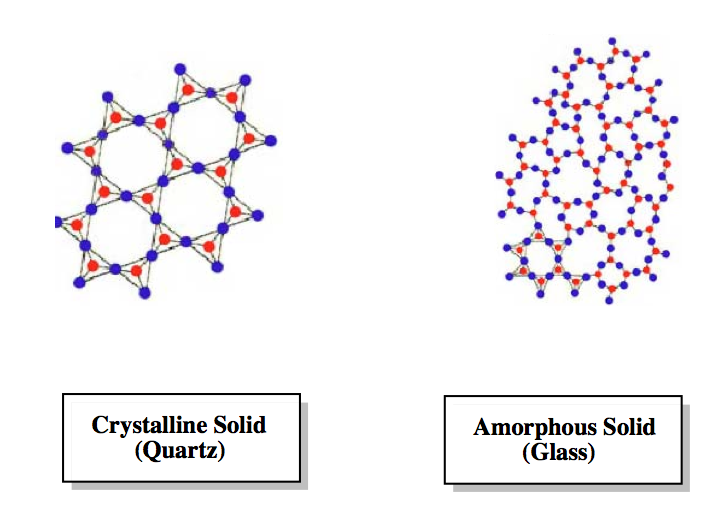When examining the particles within a crystal you may observe them tightly packed in an organized pattern. The smallest portion of which is termed the unit cell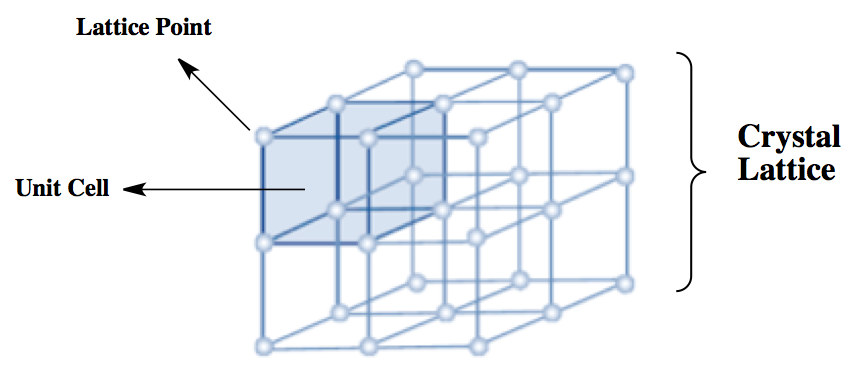###### Simple Cubic (SC) Unit Cell

There are 7 crystal systems and 14 types of unit cells that naturally occur. The simple cubic unit cell represents one of the simplest types.

Concept #2: Simple Cubic Unit Cell

The Simple Cubic Unit Cell is composed of a cube with an atom at each corner. In the Lattice Diagram each of the unit cells are combined to form a crystal lattice. In the Space Filling Unit Cell Diagram we are shown that the unit cell uses only 1/8 of each of the 8 corner atoms.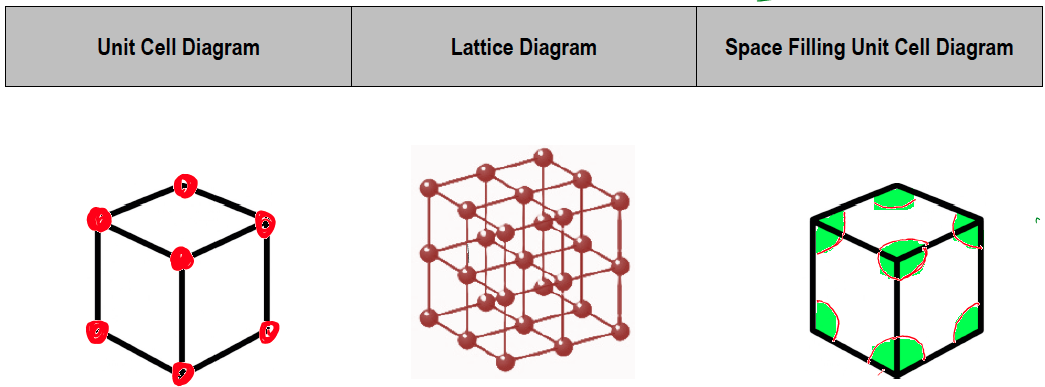###### Body-Centered Cubic (BCC) Unit Cell

The body-centered cubic unit cell is composed of a cube with one atom at each of its corners and one atom at the center of the cube.

Concept #3: Body Centered Cubic Unit Cell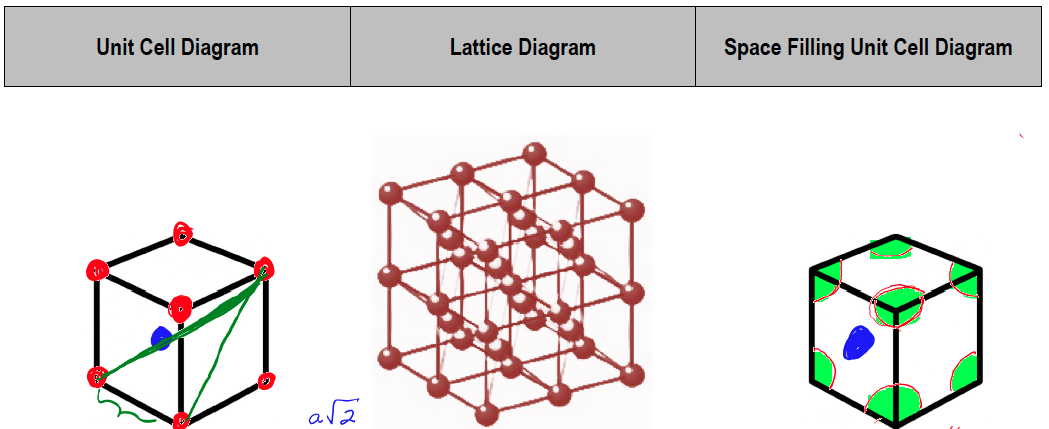###### Face-Centered Cubic (FCC) Unit Cell

The face-centered cubic unit cell is composed of a cube with one atom at each of its corners and one atom in the center of each cube face.

Concept #4: Face Centered Cubic Unit Cell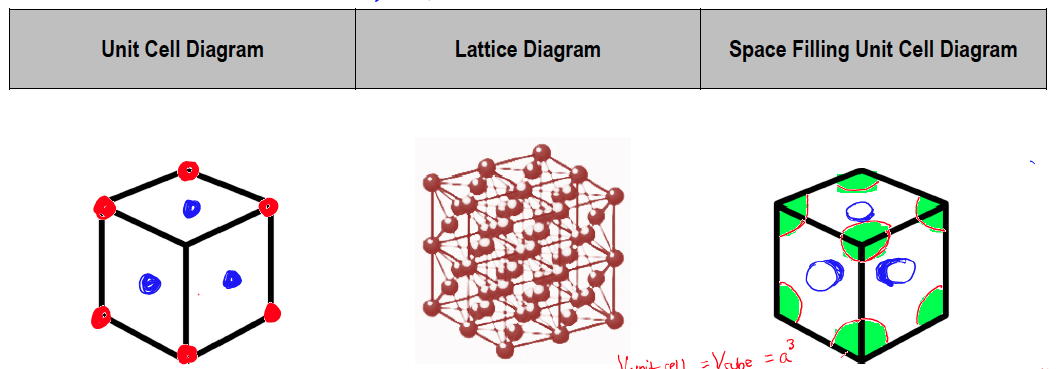###### Unit Cell Calculations

Example #1: Unit Cell Calculations 1

Example #2: Unit Cell Calculations 1

Example #3: Unit Cell Calculations 1

Example #4: Unit Cell Calculations

Example #5: Unit Cell Calculations 2

Example #6: Unit Cell Calculations 2

Example #7: Unit Cell Calculations 2

Example #8: Unit Cell Calculations 3

Example #9: Unit Cell Calculations 3

Example #10: Unit Cell Calculations 3